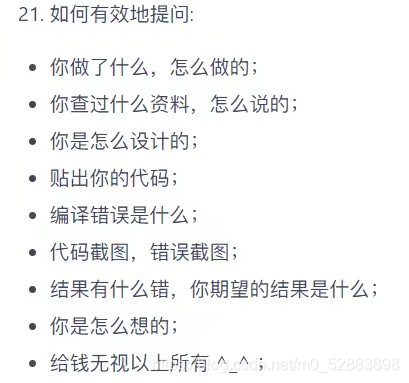# python 列表转字符串ベ布小禅@[TOC]

# 小技巧

## 问题

a = ['a', 'b', 'c', 'd']c = ''　　# 如何讲a中的字符串拼接到c中c='abcd'

## 寻求帮助## 灵光一现

a = ['a', 'b', 'c', 'd']c = a.replace('[','').replace(']','').replace(',','')replace ("'",'')

## 另一种简单方法？

a = 'abc'c = '*'.jion(a)print(c)# 结果是：a*b*c

## 确实是简单的方法！

a = ['a', 'b', 'c', 'd']c = ''.join(a)print(c)　　# 结果是：abcd

# 结语## 评论